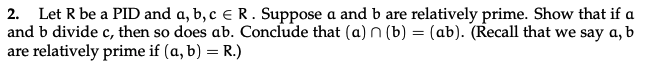Home / Answered Questions / Other / 2-let-r-be-a-pid-and-a-b-c-er-suppose-a-and-b-are-relatively-prime-show-that-if-a-and-b-divide-c-the-aw974

# (Solved): 2. Let R Be A PID And A, B, C ER. Suppose A And B Are Relatively Prime. Show That If A And B Divide ...2. Let R be a PID and a, b, c ER. Suppose a and b are relatively prime. Show that if a and b divide c, then so does ab. Conclude that (a) n (b) = (ab). (Recall that we say a, b are relatively prime if (a, b) = R.)

We have an Answer from Expert